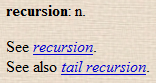# Recursive decent parsing [sic]

Someone pointed out that DecentURL didn’t detect recursive redirects, so I fixed the problem … by implementing a “recursive decent parser”.

Sorry, couldn’t help myself. :-) This blog entry does have a better point.If you’re like me and learned to program by means other than a great and glorious Computer Science course, you didn’t learn about recursive descent parsers till late in life. At least, I didn’t.

I knew about grammars and had seen many a BNF grammar, but what I didn’t know was that a BNF grammar pretty much is the parser. Take a grammar, make a few syntactic changes, and you have a parsing program.

The fact that it’s defined recursively means it automatically takes care of precedence and storing intermediate values — you get all that for free. Easy when you know how, but not immediately obvious, apparently not even to compiler writers early on in the history of computing (search down to “A little philosophy” in Let’s Build A Computer, Part IV).

Anyway, below is some sample C code. Save it out as `calc.c`, compile it, and you’ll have a simple expression calculator that’ll solve things like `4*(5+5)+2` at the drop of a hat. Note that you can use `getc()` and `ungetc()` for the look-ahead, but it’s typical for simple parsers to use a global “current char” variable, which I’ve called simply `c`:

``````/* Simple expression parser and calculator

The grammar in BNF-ish notation
-------------------------------
expression:  ['+'|'-'] term ['+'|'-' term]*
term:        factor ['*'|'/' factor]*
factor:      '(' expression ')' | number
number:      digit [digit]*

*/

#include <stdio.h>
#include <stdlib.h>
#include <ctype.h>

int c;
int expression(void);

void error(char *msg) {
puts(msg);
exit(1);
}

void next(void) {
c = getchar();
if (c==EOF) error("char expected");
}

int number(void) {
int n;

if (!isdigit(c)) error("digit expected");
n = 0;
do {
n = n*10 + c-'0';
next();
} while (isdigit(c));
return n;
}

int factor(void) {
int n;

if (c=='(') {
next();
n = expression();
if (c!=')') error(") expected");
next();
} else
n = number();
return n;
}

int term(void) {
int op, n, m;

n = factor();
while ((op=c)=='*' || op=='/') {
next();
m = factor();
n = op=='*' ? n*m : n/m;
}
return n;
}

int expression(void) {
int sign, op, n, m;

if ((sign = c=='-') || c=='+') next();
n = term();
if (sign) n = -n;
while ((op=c)=='+' || op=='-') {
next();
m = term();
n = op=='+' ? n+m : n-m;
}
return n;
}

int main(void) {
next();
printf("%d\n", expression());
return 0;
}
``````

6 November 2007 by Ben    3 comments

Ben 7 Nov 2007, 18:10 link

See also the Forth version, `calc.fs`. Use something like Gforth to run it.

angel 8 Jun 2008, 23:56 link

Hi Ben, (-200)*(500/23)+123-90/2+(40) gives -4082. Why?

Ben 9 Jun 2008, 07:29 link

Hi angel, isn’t that correct? 500/23 is 21, and -200*21 = -4200. That plus 123-45+40 = -4082.

We reserve the right to edit or remove your comment if it’s spammy, offensive, unintelligible, or if you don’t give a valid email address. :-)